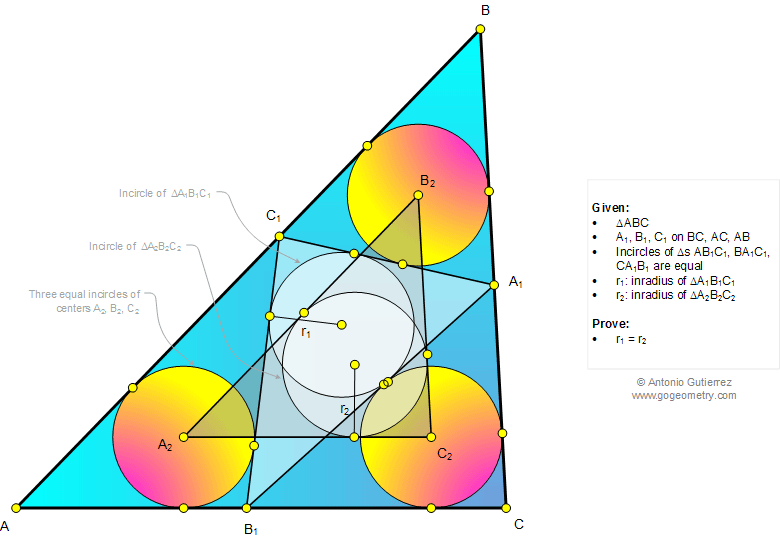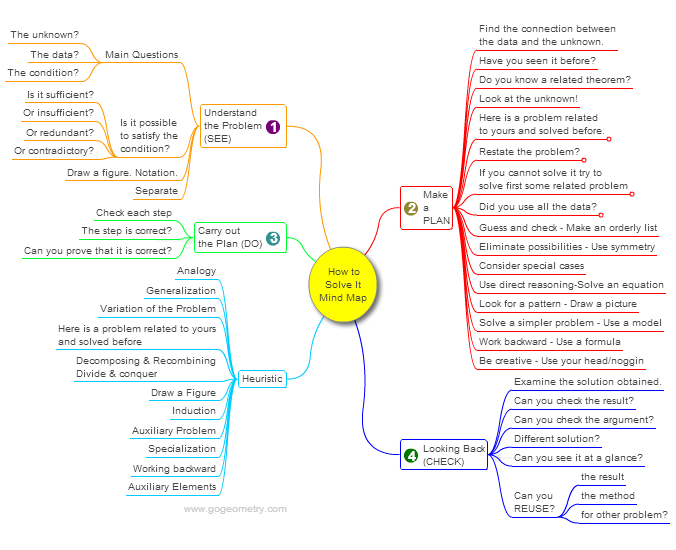# Online Math: Geometry Problem 1036: Triangle, Three equal Incircles, Tangent lines, Inradius, Equal Inradii. Level: School, College.

< PREVIOUS PROBLEM  |  NEXT PROBLEM >

 The figure below shows a triangle ABC where A1, B1, and C1 are on BC, AC, and AB, respectively, so that the incircles of triangles AB1C1, BA1C1, and CA1B1 are equal. Prove that the inradius of triangle A1B1C1 is equal to the inradius of triangle A2B2C2.## Mind Map: George Pólya's 1945 book, "How to Solve It"

George Pólya's 1945 book "How to Solve It, A new aspect of Mathematical Method", is a book describing methods of problem solving. It suggests the following steps when solving a mathematical problem: (1) First, you have to understand the problem. (2) After understanding, then make a plan. (3) Carry out the plan. (4) Look back on your work. How could it be better?
A mind map is a diagram used to visually organize information, knowledge, concepts or ideas.Home | SearchGeometry | Problems | All Problems | Open Problems | Visual Index | 10 Problems | Problems Art GalleryArt | 1031-1040 | Triangle | Circle | Incircle | Areas | Triangle area | Perimeter | Tangent Line | Email | by Antonio Gutierrez

Add a solution to the problem 1036
Last updated: Sep 19, 2014